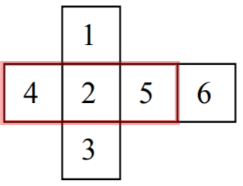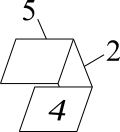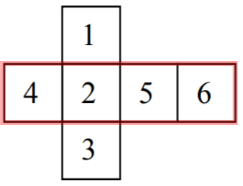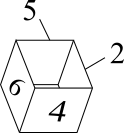#### You may also like### Trice

ABCDEFGH is a 3 by 3 by 3 cube. Point P is 1/3 along AB (that is AP : PB = 1 : 2), point Q is 1/3 along GH and point R is 1/3 along ED. What is the area of the triangle PQR?### Nine Colours

Can you use small coloured cubes to make a 3 by 3 by 3 cube so that each face of the bigger cube contains one of each colour?### Marbles in a Box

How many winning lines can you make in a three-dimensional version of noughts and crosses?

# Multiplication Cube

##### Age 11 to 14 Short Challenge Level:

The 4, 5 and 6 will be involved in the largest possible products, so we can begin by seeing whether they touch.

The 4 and the 5 do not touch, as shown in the diagram below, which shows part of the folded net.The 6 touches the 4 and the 5, as shown.This means that the greatest product must include the 6 and the 5 or the 6 and the 4.

Clearly it will include the 3, not the 1, and so it will be 6$\times$5$\times$3 = 90.
You can find more short problems, arranged by curriculum topic, in our short problems collection.